# Drawing some kinds of line in triangle

18 de Jun de 2013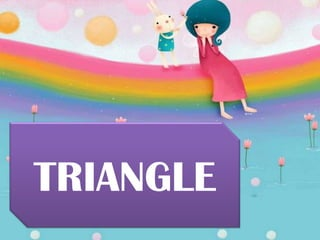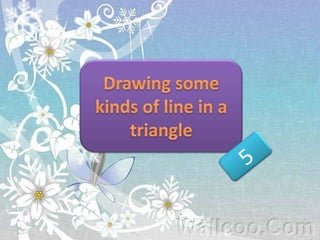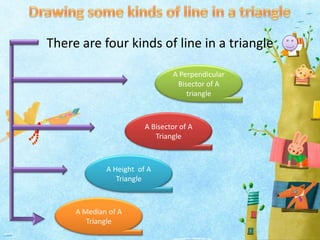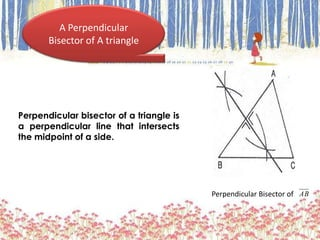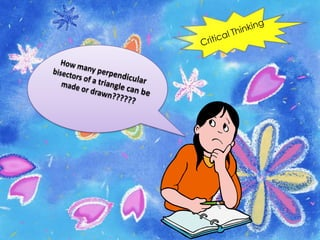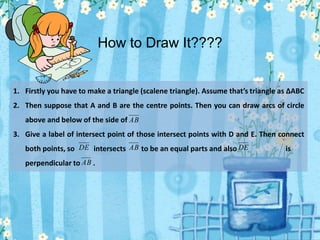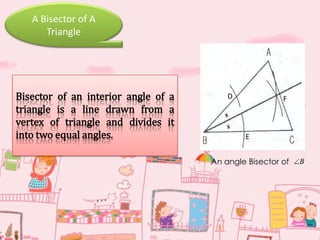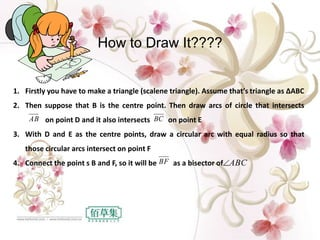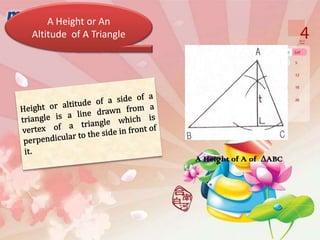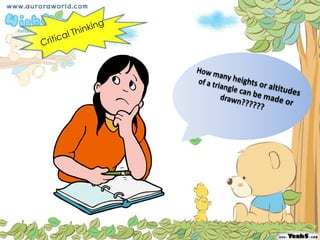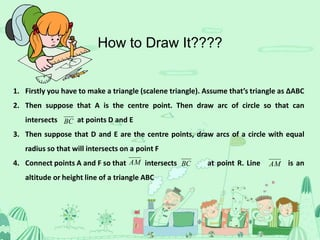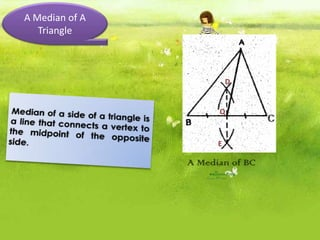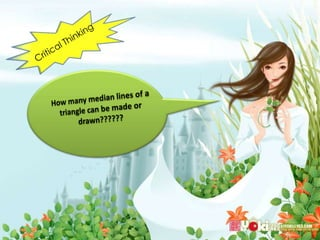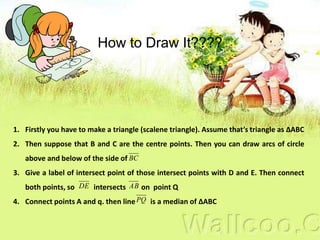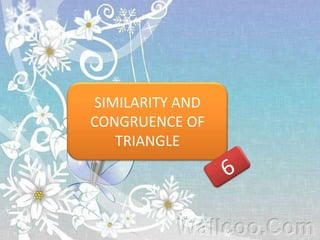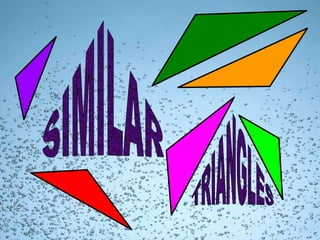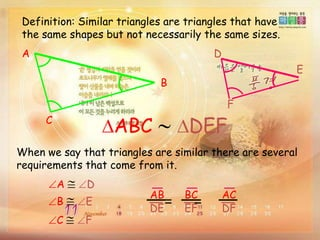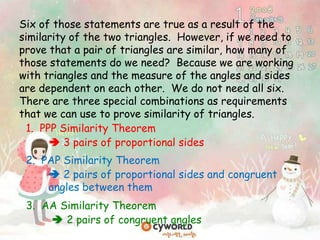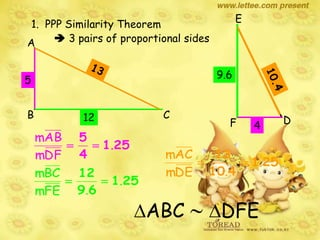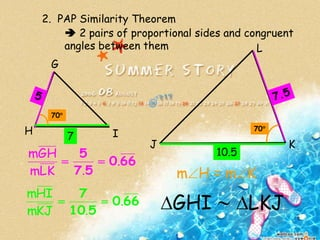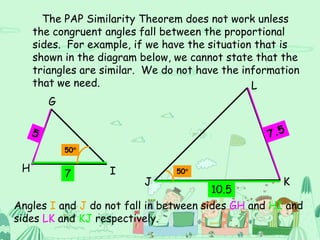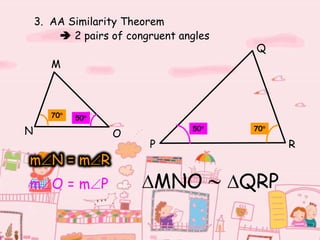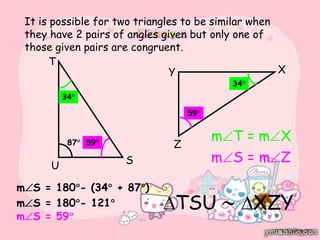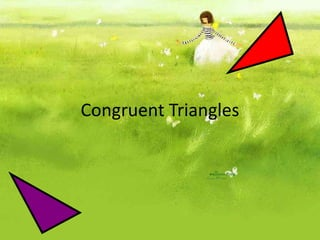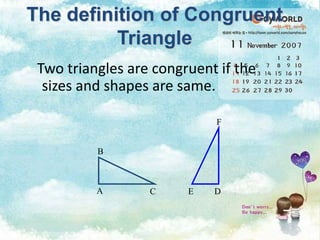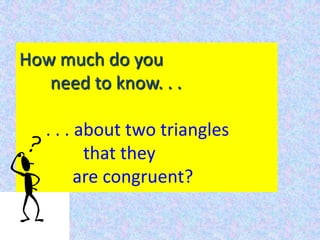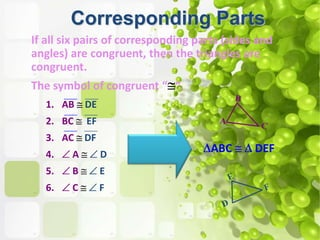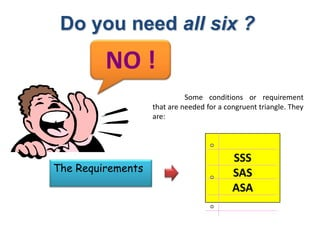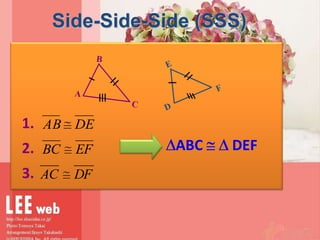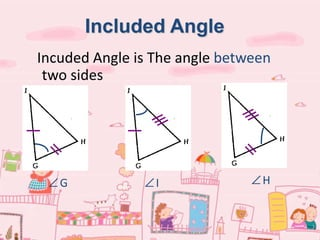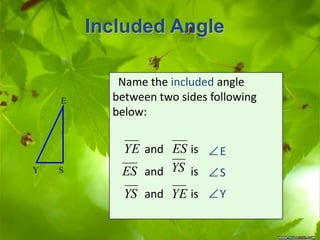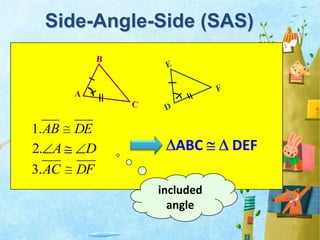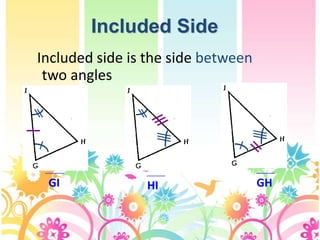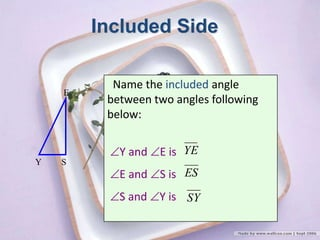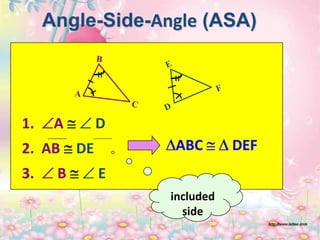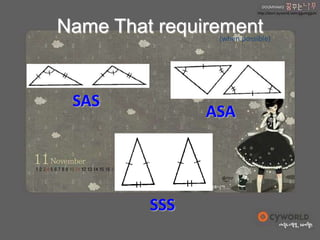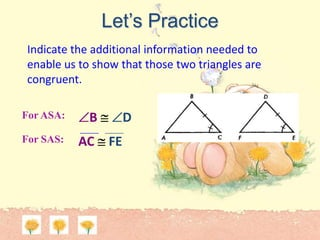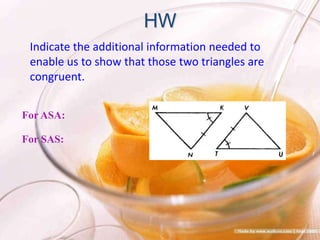1 de 40

### Drawing some kinds of line in triangle

• 3. There are four kinds of line in a triangle A Perpendicular Bisector of A triangle A Bisector of A Triangle A Height of A Triangle A Median of A Triangle
• 4. A Perpendicular Bisector of A triangle Perpendicular Bisector of AB Perpendicular bisector of a triangle is a perpendicular line that intersects the midpoint of a side.
• 6. 1. Firstly you have to make a triangle (scalene triangle). Assume that’s triangle as ∆ABC 2. Then suppose that A and B are the centre points. Then you can draw arcs of circle above and below of the side of 3. Give a label of intersect point of those intersect points with D and E. Then connect both points, so intersects to be an equal parts and also is perpendicular to . AB DE AB DE AB How to Draw It????
• 7. A Bisector of A Triangle An angle Bisector of B Bisector of an interior angle of a triangle is a line drawn from a vertex of triangle and divides it into two equal angles. D E F
• 9. 1. Firstly you have to make a triangle (scalene triangle). Assume that’s triangle as ∆ABC 2. Then suppose that B is the centre point. Then draw arcs of circle that intersects on point D and it also intersects on point E 3. With D and E as the centre points, draw a circular arc with equal radius so that those circular arcs intersect on point F 4. Connect the point s B and F, so it will be as a bisector of AB BF BC ABC How to Draw It????
• 10. A Height or An Altitude of A Triangle A Height of A of ∆ABC
• 12. 1. Firstly you have to make a triangle (scalene triangle). Assume that’s triangle as ∆ABC 2. Then suppose that A is the centre point. Then draw arc of circle so that can intersects at points D and E 3. Then suppose that D and E are the centre points, draw arcs of a circle with equal radius so that will intersects on a point F 4. Connect points A and F so that intersects at point R. Line is an altitude or height line of a triangle ABC BC AMBCAM How to Draw It????
• 13. A Median of A Triangle A Median of BC E D Q
• 15. 1. Firstly you have to make a triangle (scalene triangle). Assume that’s triangle as ∆ABC 2. Then suppose that B and C are the centre points. Then you can draw arcs of circle above and below of the side of 3. Give a label of intersect point of those intersect points with D and E. Then connect both points, so intersects on point Q 4. Connect points A and q. then line is a median of ∆ABC BC DE AB PQ How to Draw It????
• 18. Definition: Similar triangles are triangles that have the same shapes but not necessarily the same sizes. A C B D F E ABC DEF When we say that triangles are similar there are several requirements that come from it. A D B E C F AB DE BC EF AC DF = =
• 19. 1. PPP Similarity Theorem  3 pairs of proportional sides Six of those statements are true as a result of the similarity of the two triangles. However, if we need to prove that a pair of triangles are similar, how many of those statements do we need? Because we are working with triangles and the measure of the angles and sides are dependent on each other. We do not need all six. There are three special combinations as requirements that we can use to prove similarity of triangles. 2. PAP Similarity Theorem  2 pairs of proportional sides and congruent angles between them 3. AA Similarity Theorem  2 pairs of congruent angles
• 20. 1. PPP Similarity Theorem  3 pairs of proportional sidesA B C E F D 251 4 5 . DFm ABm 251 69 12 . .FEm BCm 251 410 13 . .DEm ACm 5 4 12 9.6 ABC DFE
• 21. 2. PAP Similarity Theorem  2 pairs of proportional sides and congruent angles between them G H I L J K 660 57 5 . .LKm GHm 660 510 7 . .KJm HIm 7 10.5 70 70 m H = m K GHI LKJ
• 22. The PAP Similarity Theorem does not work unless the congruent angles fall between the proportional sides. For example, if we have the situation that is shown in the diagram below, we cannot state that the triangles are similar. We do not have the information that we need. G H I L J K 7 10.5 50 50 Angles I and J do not fall in between sides GH and HI and sides LK and KJ respectively.
• 23. 3. AA Similarity Theorem  2 pairs of congruent angles M N O Q P R 70 70 50 50 m N = m R m O = m P MNO QRP
• 24. It is possible for two triangles to be similar when they have 2 pairs of angles given but only one of those given pairs are congruent. 87 34 34 S T U XY Z m T = m X m S = 180 - (34 + 87 ) m S = 180 - 121 m S = 59 m S = m Z TSU XZY 59 5959 34 34
• 26. Two triangles are congruent if the sizes and shapes are same. The definition of Congruent Triangle A C B DE F
• 27. How much do you need to know. . . . . . about two triangles that they are congruent?
• 28. If all six pairs of corresponding parts (sides and angles) are congruent, then the triangles are congruent. The symbol of congruent “ “. Corresponding Parts ABC DEF 1. AB DE 2. BC EF 3. AC DF 4. A D 5. B E 6. C F
• 29. Do you need all six ? NO ! SSS SAS ASA Some conditions or requirement that are needed for a congruent triangle. They are: The Requirements
• 31. Incuded Angle is The angle between two sides Included Angle G I H
• 32. Included Angle SY E Name the included angle between two sides following below: and is and is and is E S Y YE ES YSES YS YE
• 34. Included side is the side between two angles Included Side GI HI GH
• 35. Name the included angle between two angles following below: Y and E is E and S is S and Y is Included Side SY E YE ES SY
• 36. Angle-Side-Angle (ASA) 1. A D 2. AB DE 3. B E ABC DEF included side
• 38. Let’s Practice Indicate the additional information needed to enable us to show that those two triangles are congruent. For ASA: For SAS: B D AC FE
• 39. HW For ASA: For SAS: Indicate the additional information needed to enable us to show that those two triangles are congruent.